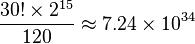# Alexander's Star facts for kids

Kids Encyclopedia Facts
TypePuzzle Adam Alexander Ideal Toy Company United States 1982–present

Alexander's Star is a puzzle similar to the Rubik's Cube, in the shape of a great dodecahedron.

## Description

The puzzle has 30 moving pieces, which rotate in star-shaped groups of five around its outermost vertices. The purpose of the puzzle is to rearrange the moving pieces so that each star is surrounded by five faces of the same color, and opposite stars are surrounded by the same color. This is equivalent to solving just the edges of a six-color Megaminx. The puzzle is solved when each pair of parallel planes is made up of only one colour. To see a plane, however, one has to look "past" the five pieces on top of it, all of which could/should have different colours than the plane being solved.

If considering the pentagonal regions as faces, like in the great dodecahedron represented by Schläfli symbol {5,5/2}, then the requirement is for all faces to be monochrome (same color) and for opposite faces to share the same color.

The puzzle does not turn smoothly, due to its unique design.

## Permutations

There are 30 edges, each of which can be flipped into two positions, giving a theoretical maximum of 30!×230 permutations. This value is not reached for the following reasons:

1. Only even permutations of edges are possible, reducing the possible edge arrangements to 30!/2.
2. The orientation of the last edge is determined by the orientation of the other edges, reducing the number of edge orientations to 229.
3. Since opposite sides of the solved puzzle are the same color, each edge piece has a duplicate. It would be impossible to swap all 15 pairs (an odd permutation), so a reducing factor of 214 is applied.
4. The orientation of the puzzle does not matter (since there are no fixed face centers to serve as reference points), dividing the final total by 60. There are 60 possible positions and orientations of the first edge, but all of them are equivalent because of the lack of face centers.

This gives a total of$\frac{30!\times 2^{15}}{120} \approx 7.24\times 10^{34}$ possible combinations.

The precise figure is 72 431 714 252 715 638 411 621 302 272 000 000 (roughly 72.4 decillion on the short scale or 72.4 quintilliard on the long scale).Alexander's Star Facts for Kids. Kiddle Encyclopedia.